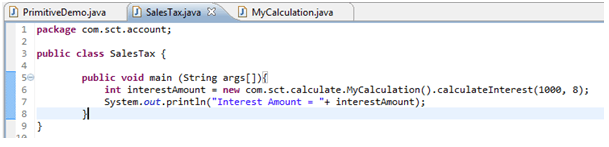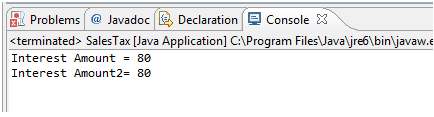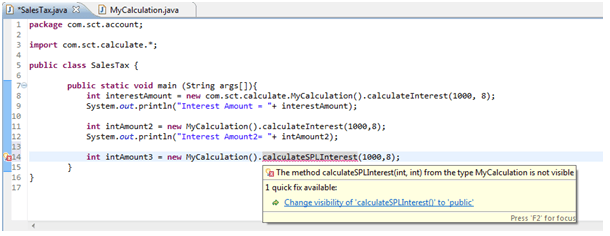# Java Packages

## Introduction

A decent sized project can have hundreds of Java classes and you need to organize them in packages (think file directories). This will allow you to categorize files, control access to your classes, and avoid potential naming conflicts. Sometimes you’ll be using third-party libraries of Java classes written in a different department or even outside your firm. To minimize the chances that package names will be the same, it’s common to use so-called reverse domain name conventions. For example, if you work for a company called SCT, which has the website sct.com, you can prefix all package names with com.sct. To place a Java class in a certain package, add the package statement at the beginning of the class (it must be the first non-comment statement in the class).For example, if the class SalesTax has been developed for the Accounting department, you can declare it as follows, which will result in creation of directory structure as below:``````packagecom.sct.account;
classSalesTax {
// the class body goes here
}
```
```

Java classes are also organized into packages and the fully qualified name of a class consists of the package name followed by the class name. For example, the full name of the Java class Double isjava.lang.Double, where java.lang is the package name.

To use a public package member from outside its package, you must do one of the following:

• Refer to the member by its fully qualified name: Here no need of import statement but the code will become non-readable due to the longer statement.
`newpackageName.className().methodName( argument1, argument2); `
• Import the package member: This way we can import one class from one package. Import statement should be put after package declaration statement.
`importpackageName.className; newclassName().methodName(argument 1, argument2); `
• Import the member's entire package: This will help to import all the classes of a particular package.
```importpackageName.*;
newclassName().methodName(argument1, argument2);
```

We can put our class file on “classpath” to have global access without the need of import but it is not advisable programming way. We have earlier discussed how to create/update “classpath”. We can add our java compiled directory to classpath like below,

` [packageDir]\packageName\ClassName.java`

Lets assume our SalesTax class needs to use calculateInterest() method of class “MyCalculation” declared in package called “com.sct.calculate”.

``````package com.sct.calculate;
public class MyCalculation
{
//This method calculate interst
public int calculateInterest(int amount, int rate)
{
int intrerstAmount = amount * rate/100;
return intrerstAmount;
}
}
```
```

We can call calculateInterest() method in two way.

1. Calling with the fully qualified name: As seen below we are calling the method with full path.2. package import and then directly calling the method: Here we have added line number 3 which is import statement and line number 11 will be doing exactly same as line number 8.

``````package com.sct.account;
import com.sct.calculate.*;
public class SalesTax {
public static void main (String args[]){
int interestAmount = new com.sct.calculate.MyCalculation().calculateInterest(1000, 8);
System.out.println("Interest Amount = "+ interestAmount);
int intAmount2 = new MyCalculation().calculateInterest(1000,8);
System.out.println("Interest Amount2= "+ intAmount2);
}
}
```
```

Output of above SalesTax class will be as below

Sample Output:If you need to import several classes from the same package, use the wildcard in the import statement rather than listing each of the classes on a separate line as shown below,

``````
package com.sct.account;
import com.sct.calculate.*;
public class SalesTax
{
public static void main (String args[])
{
int interestAmount = new com.sct.calculate.MyCalculation().calculateInterest(1000, 8);
System.out.println("Interest Amount = "+ interestAmount);
int intAmount2 = new MyCalculation().calculateInterest(1000,8);
System.out.println("Interest Amount2= "+ intAmount2);
}
}
```
```

Package is mainly used to implement Encapsulation principle of Java OOP. Lets take an example. We have requirement to have one method calculateSPLInterest() accessible only in same package ie. “com.sct.calculate”. This might be for bank’s own employee interest calculation. So our “MyCalculation” class will look like below

``````package com.sct.calculate;
public class MyCalculation {
//This method calculate interst
public int calculateInterest(int amount, int rate){
int intrerstAmount = amount * rate/100;
return intrerstAmount;
}
//This is Special Interst Calculation
protected int calculateSPLInterest (int amount, int rate){
int intrerstAmount = (amount * rate/100) + 100;
return intrerstAmount;
}
}
```
```

While trying to access this method from outside package, compiler will give error as shown in below screen. This way we can hide particular class member implementation outside package.Here the programs are small and use a limited number of classes, you might find that you don’t need to explore packages at all. But as you begin creating more sophisticated projects with many classes related to each other by inheritance, you might discover the benefit of organizing them into packages.

Packages are useful for several broad reasons:

• They enable you to organize your classes into units. Just as you have folders or directories on your hard disk to organize your files and applications, packages enable you to organize your classes into groups so that you use only what you need for each program.
• They reduce problems with conflicts about names. As the number of Java classes grows, so does the likelihood that you’ll use the same class name as another developer, opening up the possibility of naming clashes and error messages if you try to integrate groups of classes into a single program. Packages provide a way to refer specifically to the desired class, even if it shares a name with a class in another package.
• They enable you to protect classes, variables, and methods in larger ways than on a class-by-class basis, as you learned today. You learn more about protections with packages later.
• Packages can be used to uniquely identify your work.

Summary

• Java package is a way to organize the classes in a large project and it also helps to in encapsulation implementation.
• To access code residing outside the current package, either import the class or use the fully qualified class name.
﻿